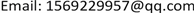1三峡大学计算机与信息学院，湖北 宜昌

2三峡大学理学院，湖北 宜昌1. 研究背景

2. 问题分析

3. 建立模型3.1. 轨迹数据预处理

3.2. 去除数据冗余

3.3. 样条插值

Δ : a = x 0 < x 1 < ⋯ < x n = b

3.4. 噪点过滤

1) 均值过滤。如果某一个异常点对于它前后两个观测点位置偏移量极大时，可以采用均值过滤的方法，使车辆在单位时间内的位移距离更加合理。第K个点的位置估值可以表示为：

P K = P k − 1 + P k + 1 2

2) 卡尔曼滤波过滤。卡尔曼过滤根据测量值和运动趋势得到轨迹估值，卡尔曼过滤基于轨迹符合线性模型的假设，根据运动物体转移概率矩阵的关系，对异常值的采集点预测并行校正  。

X ( k + 1 ) = A ( k ) X ( k ) + T ( k ) W (k)

Z ( k ) = H ( k ) X ( k ) + V (k)

M = E [ ( x i − x ′ i ) ( x i − x ′ i ) T ]

X ( k + 1 , k ) = A ( k ) X ( k , k ) Z ( k + 1 , k ) = H ( k ) X ( k + 1 , k )

3) 基于启发式的异常检测模型。

V K = S t

3.5. 基于隐马尔科夫的地图匹配算法

Step 1：确定参数：

HMM的5个基本因素，由状态变量数，观测变量数，状态转移矩阵，观测概率矩阵，初始状态概率向量组成  。

1) 确定观察变量。从车联网采集的数据中得到的位置信息(包括经度，纬度)；

2) 隐常状态。此时在路网上的运输车的实际所在位置；

3) 观测概率。观测经纬度离旁边道路近，那么真实点在这个路段的概率越大  ，假设服从高斯分布设有：

p ( z t | r i ) = 1 2 π σ z e − 0.5 ( ‖ z t − x t , i ‖ σ z ) 2

σ z = 1.4826 m e d i a n t ( ‖ z t − x t , i ‖ )

4) 状态转移概率。在两个时间点候选路段的转换概率：

p ( d t ) = 1 β e − d t / β

d t = | ‖ z t − z t + 1 ‖ − ‖ x t , i − x t + 1 , i ‖ | ， β = 1 ln ( 2 ) m e d i a n t (dt)

5) 状态转移矩阵A：

a i j = P ( q t + 1 = r j | q t = r i ) = e − r i j

r j 是时刻t + 1时所在路段， r i 是时刻t所在路段， r i j 有三种状态，当 r i j 为1时，相邻， r i j 为为无穷大时，不相邻， r i j 等于0时，在同一路段。

Step 2：Viterbi算法求解

δ t ( i ) = max P ( i t = i , i t − 1 , ⋯ , i 1 , o t , ⋯ , o 1 | λ ) δ t + 1 ( i ) = max i 1 , ⋯ , i t P ( i t = i , i t − 1 , ⋯ , i 1 , o t , ⋯ , o 1 | λ )

ψ t ( i ) = arg max [ δ t − 1 ( j ) a i j ]

4. 实验求解

5. 总结